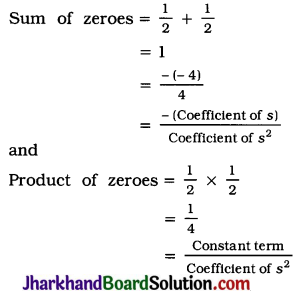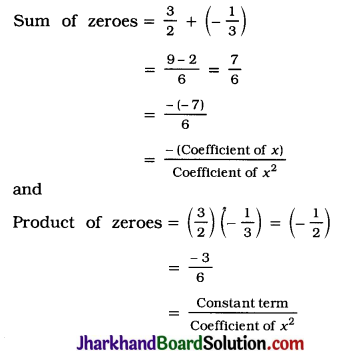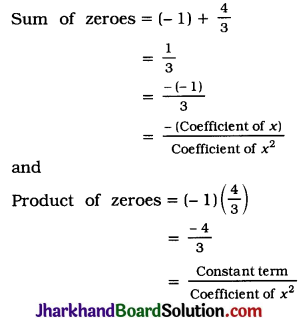# JAC Class 10 Maths Solutions Chapter 2 Polynomials Ex 2.2

Jharkhand Board JAC Class 10 Maths Solutions Chapter 2 Polynomials Ex 2.2 Textbook Exercise Questions and Answers.

## JAC Board Class 10 Maths Solutions Chapter 2 Polynomials Exercise 2.2

Question 1.
Find the zeroes of the following quadratic polynomials and verify the relationship between the zeroes and the coefficients :
1. x2 – 2x – 8
2. 4s2 – 4s + 1
3. 6x2 – 3 – 7x
4. 4u2 + 8u
5. t2 – 15
6. 3x2 – x – 4
Solution:
1. x2 – 2x – 8 = x2 – 4x + 2x – 8
= x(x – 4) + 2(x – 4)
= (x – 4)(x + 2)
So, the value of x2 – 2x – 8 is zero, when
x – 4 = 0 or x + 2 = 0, i.e., when x = 4 or x = -2.
Hence, the zeroes of polynomial x2 – 2x – 8 are 4 and -2.
Now,
Sum of zeroes = 4 + (-2)
= 2
= $$\frac{-(-2)}{1}$$
= $$\frac{-(\text { Coefficient of } x)}{\text { Coefficient of } x^2}$$
and Product of zeroes = (4) (-2)
= -8
= $$\frac{-8}{1}$$
= $$\frac{\text { Constant term }}{\text { Coefficient of } x^2}$$

2. 4s2 – 4s + 1 = 4s2 – 2s – 2s + 1
= 2s (2s – 1) -1 (2s – 1)
= (2s – 1)(2s – 1)
So, the value of 4s2 – 4s + 1 is zero, when $$\frac{1}{2}$$ or
s = $$\frac{1}{2}$$
2s – 1 = 0 or 2s – 1 = 0, i.e., when s = 2
Hence, the zeroes of polynomial 4s2 – 4s + 1 are $$\frac{1}{2}$$ and $$\frac{1}{2}$$ (both equal).
Now,3. 6x2 – 3 – 7x = 6x2 – 9x + 2x – 3
= 3x(2x – 3) + 1(2x – 3)
= (2x – 3)(3x + 1)
So, 6x2 – 3 – 7x = 0 when 2x – 3 = 0 or 3x + 1 = 0,
i.e., when x = $$\frac{3}{2}$$ or x = –$$\frac{1}{3}$$.
Hence, the zeroes of polynomial 6x2 – 3 – 7x are $$\frac{3}{2}$$ and –$$\frac{1}{3}$$.
Now,4. 4u2 + 8u = 4u (u + 2)
So, 4u2 + 8u = 0 when 4u = 0 or u + 2 = 0,
i.e., when u = 0 or u = -2.
Hence, the zeroes of polynomial 4u2 + 8u are 0 and -2.
Now,
Sum of zeroes = 0 + (-2)
= -2
= $$\frac{-(8)}{4}$$
= $$\frac{-(\text { Coefficient of } u)}{\text { Coefficient of } u^2}$$
and
Product of zeroes = (0)(-2) = 0
= $$\frac{0}{4}$$
= $$\frac{\text { Constant term }}{\text { Coefficient of } u^2}$$
Note: In polynomial
4u2 + 8u = 4u2 + 8u + 0, the constant term is 0.

5. t2 – 15 = (t)2 – ($$\sqrt{15}$$)2
= (t + $$\sqrt{15}$$) (t – $$\sqrt{15}$$)
So, t2 – 15 = 0 when t + $$\sqrt{15}$$ = 0 or
t – 15 = 0, i.e., when t = –$$\sqrt{15}$$ or t = $$\sqrt{15}$$.
Hence, the zeroes of polynomial t2 – 15 are –$$\sqrt{15}$$ and $$\sqrt{15}$$.
Now,
Sum of zeroes = ($$-\sqrt{15}$$) + ($$\sqrt{15}$$)
= 0
= $$\frac{-0}{1}$$
= $$\frac{-(\text { Coefficient of } t)}{\text { Coefficient of } t^2}$$
and
Product of zeroes = ($$\sqrt{15}$$) ($$\sqrt{15}$$)
= -15
= $$\frac{-15}{1}$$
= $$\frac{\text { Constant term }}{\text { Coefficient of } t^2}$$
Note: In polynomial t2 – 15 = t2 + 0t – 15, the coefficient of t is 0.

6. 3x2 – x – 4 = 3x2 + 3x – 4x – 4
= 3x(x + 1) – 4(x + 1)
= (x + 1)(3x – 4)
So, 3x2 – x – 4 = 0 when x + 1 = 0 or 3x – 4 = 0, i.e., when x = -1 or x = $$\frac{4}{3}$$
Hence. -1 and $$\frac{4}{3}$$ are the zeroes of polynomial 3x2 – x – 4.
Now,Question 2.
Find a quadratic polynomial each with the given numbers as the sum and product of its zeroes respectively:
1. $$\frac{1}{4}$$, -1
2. $$\sqrt{2}$$, $$\frac{1}{3}$$
3. 0, $$\sqrt{5}$$
4. 1, 1
5. $$-\frac{1}{4}, \frac{1}{4}$$
6. 4, 1
Solution:
1. $$\frac{1}{4}$$, -1
Let the quadratic polynomial be ax2 + bx + c and its zeroes be α and β.
Then, as per given,
α + β = $$\frac{1}{4}$$ = $$\frac{-b}{a}$$
and αβ = -1 = $$\frac{c}{a}$$
If a = 4, then b = -1 and c = -4.
So, one quadratic polynomial satisfying the given condition is 4x2 – x – 4.
In general, a quadratic polynomial satisfying the given condition is k(4x2 – x – 4), where k is a non-zero real number.

2. $$\sqrt{2}$$, $$\frac{1}{3}$$
Let the quadratic polynomial be ax2 + bx + c and its zeroes be α and β.
Then, as per given,
α + β = $$\sqrt{2}$$ = $$\frac{-b}{a}$$
and αβ = $$\frac{1}{3}$$ = $$\frac{c}{a}$$
If a = 3, then b = -3$$\sqrt{2}$$ and c = 1
So, one quadratic polynomial satisfying the given condition is 3x2 – 3$$\sqrt{2}$$x + 1.
In general, a quadratic polynomial satisfying the given condition is k(3x2 – 3$$\sqrt{2}$$x + 1).
where k is a non-zero real number.

3. 0, $$\sqrt{5}$$
Let the quadratic polynomial be ax2 + bx + c and its zeroes be α and β.
Then, as per given, α + β = 0 = $$\frac{-b}{a}$$
and αβ = $$\sqrt{5}$$ = $$\frac{c}{a}$$
If a = 1, then b = 0 and c = $$\sqrt{5}$$.
So, one quadratic polynomial satisfying the given condition is x2 + $$\sqrt{5}$$.
In general, a quadratic polynomial satisfying the given condition is k(x2 + $$\sqrt{5}$$), where k is a non-zero real number.

4. 1, 1
Let the quadratic polynomial be ax2 + bx + c and its zeroes be α and β.
Then, as per given.
α + β = 1 = $$\frac{-b}{a}$$
and αβ = 1 = $$\frac{c}{a}$$
If a = 1, then b = -1 and c = 1.
So, one quadratic polynomial satisfying the given condition is x2 – x + 1.
In general, a quadratic polynomial satisfying the given condition is k(x2 – x + 1), where k is a non-zero real number.

5. $$-\frac{1}{4}, \frac{1}{4}$$
Let the quadratic polynomial be ax2 + bx + c and its zeroes be α and β.
Then, as per given,
α + β = $$-\frac{1}{4}$$ = $$\frac{-b}{a}$$
and αβ = $$\frac{1}{4}$$ = $$\frac{c}{a}$$
If a = 4, then b = 1 and c = 1.
So, one quadratic polynomial satisfying the given condition is 4x2 + x + 1.
In general, a quadratic polynomial satisfying the given condition is k(4x2 + x + 1), where k is a non-zero real number.

6. 4, 1
Let the quadratic polynomial be ax2 + bx + c and its zeroes be α and β.
Then, as per given.
α + β = 4 = $$\frac{-b}{a}$$
and αβ = 1 = $$\frac{c}{a}$$
If a = 1, then b = -4 and c = 1.
So, one quadratic polynomial satisfying the given condition is x2 – 4x + 1.
In general, a quadratic polynomial satisfying the given condition is k(x2 – 4x + 1), where k is a non-zero real number.